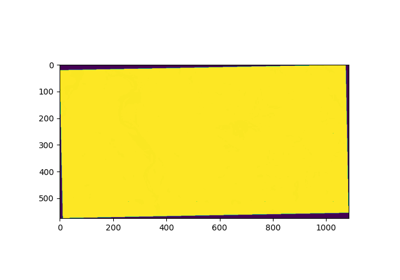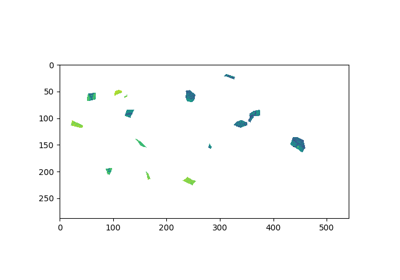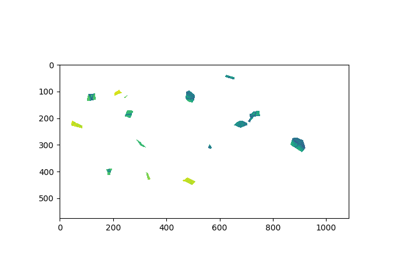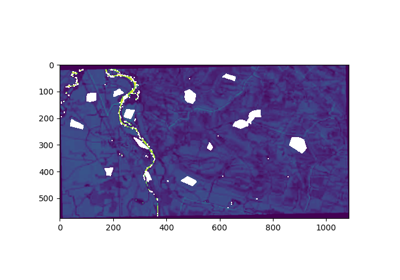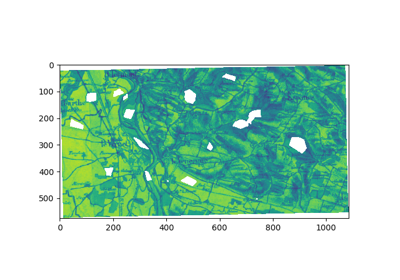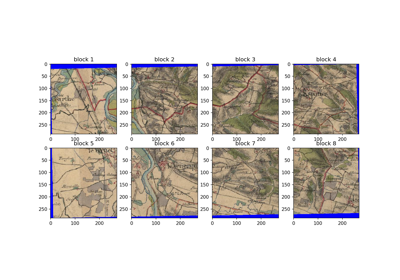# RasterMath¶

class `museotoolbox.processing.``RasterMath`(in_image, in_image_mask=False, return_3d=False, block_size=[256, 256], n_jobs=1, message='rasterMath...', verbose=True)[source]

Read one or multiple rasters per block, and perform one or many functions to one or many geotiff raster outputs. If you want a sample of your data, just call `get_random_block()`.

The default option of rasterMath will return in 2d the dataset :
• each line is a pixel with in columns its differents values in bands so masked data will not be given to this user.

If you want to have the data in 3d (X,Y,Z), masked data will be given too (using numpy.ma).

Parameters
• in_image (str.) – Path of a gdal extension supported raster.

• in_image_mask (str or False, optional (default=False)) – If str, path of the raster mask. Value masked are 0, other are considered not masked. Use `invert=True` in `museotoolbox.processing.image_mask_from_vector` to mask only what is not in polygons.

• return_3d (bool, optional (default=False)) – Default will return a row per pixel (2 dimensions), and axis 2 (bands) are columns. If `return_3d=True`, will return the block without reshape (not suitable to learn with sklearn).

• block_size (list or False, optional (default=[256,256])) – Define the reading and writing block size. First element is the number of columns, second element the number of lines per block. If False, will use the block size as defined in in_image. To define later the block_size, use custom_block_size.

• n_jobs (int, optional, (default value : 1)) – Numbers of workers or process that will work in parallel. To use if your function are very time consumming.

• message (str, optional (default='rasterMath...')) – If str, the message will be displayed before the progress bar.

• verbose (bool or int, optional (default=True)) – The higher is the int verbose, the more it will returns informations.

Examples

```>>> import museotoolbox as mtb
>>> rM = mtb.processing.RasterMath(raster)
Total number of blocks : 15
Using datatype from numpy table : int16.
Detected 1 band for function mean.
>>> rM.run()
rasterMath... [########################################]100%
Saved /tmp/test.tif using function mean
```

Methods

 `__init__`(in_image[, in_image_mask, …]) Initialize self. `add_function`(function, out_image[, …]) Add function to rasterMath. `add_image`(in_image) Add raster image. `custom_block_size`([x_block_size, y_block_size]) Define custom block size for reading and writing the raster. `custom_raster_parameters`(parameters_list) Parameters to custom raster creation. `get_block`([block_number, return_with_mask]) Get a block by its position, ordered as follow : `get_block_coords`([block_number]) Get position of a block : Return the input image(s) as an array. `get_random_block`([random_state]) Get a random block from the raster. Get raster parameters (compression, block size…) Yields each whole band as np masked array (so with masked data) Yield each block. Reshape array with at least one band. `run`([memory_size]) Execute and Write output according to given functions. `write_block`(block, idx_block, idx_func) Write a block at a position on a output image

## Examples using `museotoolbox.processing.RasterMath`¶